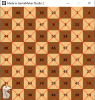# The Knight's Tour

#### Bentley

##### Member
GM Version: GM2

Summary:
The Knight's Tour is a Chess problem where you try to move the knight to every square on the Chessboard without revisiting a square. The Warnsdorf algorithm makes this complex problem simple. In this tutorial we will code an animated version of the algorithm. Click the link above to see it run.

A finished Knight's Tour showing the path of the knight.Algorithm
1. Mark the knight's square visited.
2. Look at all the valid squares the knight can move to. A valid square is both on the board and unvisited. Give each of those potential squares a score. That score is equal to how many valid moves a knight could make from the potential square.
3. Move the knight to the square with the smallest score (ties between scores are ok, just pick one of the lowest).
4. Repeat 1-3.

Tutorial:
[Create Event]
GML:
``````#macro COLS 8
#macro ROWS 8
#macro CELL_SIZE (room_width div COLS)
#macro c_light make_color_rgb(241, 194, 125)
#macro c_dark  make_color_rgb(141, 85, 36)

// move_x/y are synced arrays storing the 8 moves the knight can make
move_x = [1, 2, 2, 1, -1, -2, -2, -1];
move_y = [-2, -1, 1, 2, 2, 1, -1, -2];

// This 2D array is initialized to 0 and has two purposes:
// Boolean checks for unvisited squares and for numbering the Knight's path through the board (from 1 to 64).
for (var i = 0; i < COLS; i++)
{
for (var j = 0; j < ROWS; j++)
{
visited[i, j] = 0;
}
}

// Save the knight's position
knight_x = irandom(COLS - 1);
knight_y = irandom(ROWS - 1);

// Make the square the knight starts on a part of the tour by setting it to 1
visited[knight_x, knight_y] = 1;

[Step Event]
GML:
``````// Run the algorithm each step until 64 moves are made
{
// Find the square with the least score
var least = infinity;
var least_x = -1;
var least_y = -1;

// Loop through the moves
for (var i = 0; i < 8; i++)
{
var check_x = knight_x + move_x[i];
var check_y = knight_y + move_y[i];

// A square is valid if it's on the board and unvisited
if (is_valid(check_x, check_y))
{
// Get the square's score and if it's the smallest...
var _score = score_from_square(check_x, check_y);
if (_score < least)
{
// Save the square's (x,y) for moving the knight
// Save the square's score for further comparisions
least_x = check_x;
least_y = check_y;
least = _score;
}
}
}

// Move!
knight_x = least_x;
knight_y = least_y;

// Make this square a part of the tour by setting it to the move number (from 2-64)
}``````
score_from_square(x, y)
GML:
``````/// @func score_from_square(x, y)
/// @arg x
/// @arg y
/// @desc Return a score equal to the amount of valid moves from (x,y)

var xx = argument0;
var yy = argument1;

// Count how many squares the knight could move to if it were at (x, y)
var _score = 0;

// Loop through the moves
for (var i = 0; i < 8; i++)
{
var check_x = xx + move_x[i];
var check_y = yy + move_y[i];

// If the square we're checking is valid, increment the score
if (is_valid(check_x, check_y))
{
_score++;
}
}

return _score;``````
is_valid(x, y)
GML:
``return (inside(argument0, argument1) && !visited[argument0, argument1]);``
inside(x, y)
GML:
``return (point_in_rectangle(argument0, argument1, 0, 0, COLS - 1, ROWS - 1));``
[Draw Event]
GML:
``````draw_board();

draw_set_halign(fa_center);
draw_set_valign(fa_middle);
draw_set_color(c_black);

// Draw stuff on visited squares
for (var i = 0; i < COLS; i++)
{
for (var j = 0; j < ROWS; j++)
{
if (visited[i, j])
{
var x1 = i * CELL_SIZE;
var y1 = j * CELL_SIZE;
var x2 = (i + 1) * CELL_SIZE;
var y2 = (j + 1) * CELL_SIZE;

// Cross it out
draw_line_color(x2, y1, x1, y2, c_red, c_red);
draw_line_color(x1, y1, x2, y2, c_red, c_red);

// Draw the move number
draw_text(x1 + CELL_SIZE / 2, y1 + CELL_SIZE / 2, visited[i, j]);
}
}
}

// Draw the knight
draw_sprite_ext(s_knight, 0, knight_x * CELL_SIZE + CELL_SIZE / 2, knight_y * CELL_SIZE + CELL_SIZE / 2, 0.75, 0.75, 0, c_white, 0.66);``````
draw_board()
GML:
``````for (var i = 0; i < COLS; i++)
{
for (var j = 0; j < ROWS; j++)
{
// Alternate light and dark
var color = ((i mod 2 == 0 && j mod 2 == 0) || (i mod 2 != 0 && j mod 2 != 0) ? c_light : c_dark);

// Draw the square
draw_set_color(color);
draw_rectangle(i * CELL_SIZE, j * CELL_SIZE, (i + 1) * CELL_SIZE, (j + 1) * CELL_SIZE, false);
draw_set_color(-1);
}
}``````
I like this algorithm because it shows how an easy approach can solve a difficult problem. And if you really want to see why this algorithm is so good at this problem, try solving it with recursive backtracking.
I could only solve the Knight's Tour with recursive backtracking on a 4x4 board. And even then the knight must start in a corner (so that only 2 initial moves are possible).
Thanks for checking it out.

Last edited: